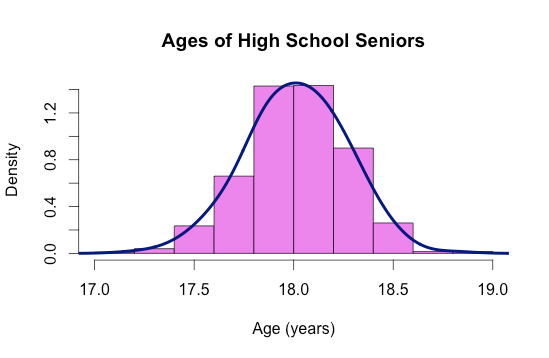1
2
3
4
5
#

R - Histograms

Histograms are graphs that represent the distribution of data using bars. Each bar (called a “bin”) represents one interval of data. Smaller intervals means more bins are used in the graph, while larger intervals means less bins are used. The height of each bar corresponds to how frequently data that falls in that bin (within that interval) appears.

Constructing Histograms in R

For a set of data x, the basic command to create a histogram is:

hist(x)

 R Code   x=c(10, 10, 12, 12, 8, 4, 7, 8, 9, 10) hist(x) Output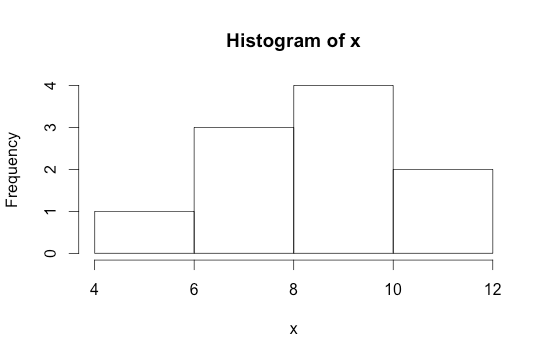Bins

To define the number of bins in your histogram:

hist(x, breaks=numberofbins)
 R Code   x=c(10,10,12,12,8,4,7,8,9,10) hist(x, breaks = 8) Output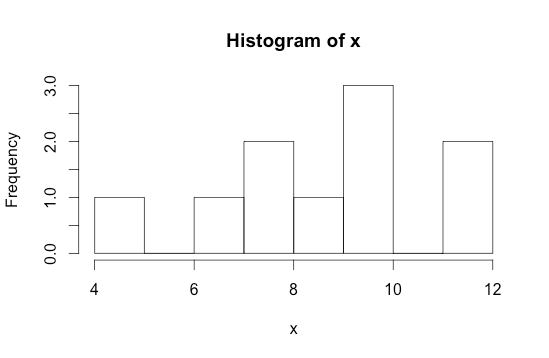To change the y-axis from frequency to probability:

hist(x, prob=T)

 R Code   a = rnorm(1000, 100, 5) hist(a, prob = T) Output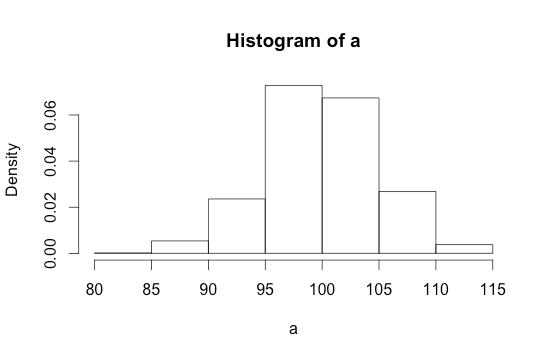lines(density(x, bw=number)

 R Code   hist(a, prob = T) lines(density(a, bw=1.5), col= “blue”) Output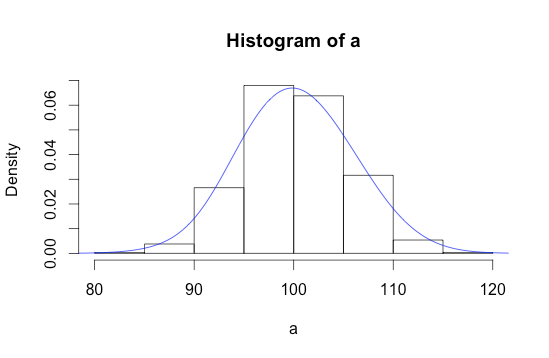Example 1:

 RStudio Code   hist(Orange\$circumference, xlab = "Circumference (mm)", ylab = "Number of Trees", main = "Circumference of Orange Trees", col = "orange", breaks=10) Output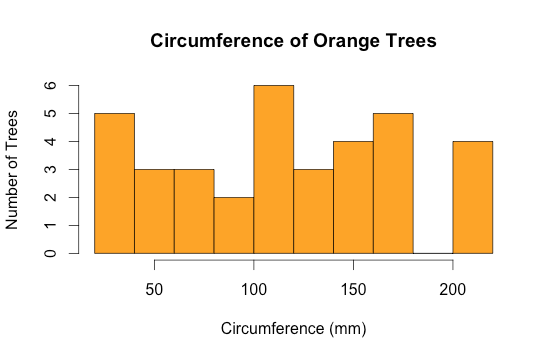Example 2:

 RStudio Code   x=c(25,25,25,25,25,20,45,45,30,50,15,30,30,30, 30,25,50, 25,25,30,25,20,30,30,15) hist(x, xlab= “Time (minutes)”, ylab= “Number of People”, main= “Time it Takes to Get to School”, col= “cyan1”, breaks=7) Output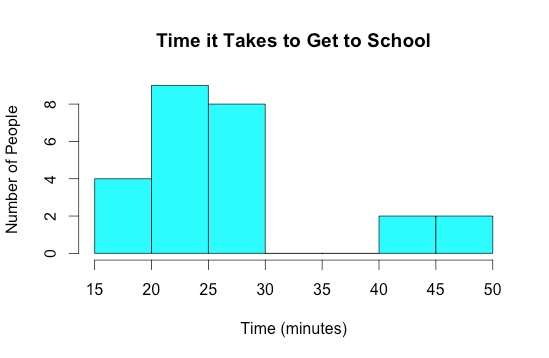Example 3:

 RStudio Code   hist(a, xlab = "Age (years)", prob = T, main = "Ages of High School Seniors", col = "violet") lines(density(a, bw=0.10), col="navy", lwd = 4) Output